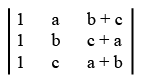# ISRO Scientist or Engineer Mechanical 2006

Instructions

For the following questions answer them individually

Question 71

# A unit vector perpendicular to the vectors $$\overrightarrow{a} = 2i - 3j + k$$ and $$\overrightarrow{b} = i + j - 2k,$$ isQuestion 72

# The region of the z plane for which $$|\frac {z - a}{z + a}| = (Re a \neq 0)$$ isQuestion 73

# If $$\alpha, \beta, \gamma$$ are the roots of equations $$X^3 + Px^2 + qx + p = 0$$, Then the value of $$\tan^{-1} \alpha + \tan^{-1} \beta + \tan^{-1} \gamma$$ isQuestion 74

# The value of the determinantisQuestion 75

# The value of $$\int_{0}^{1} \int_{0}^{1} \frac{dx dy} {\sqrt{ (1 -x^2)(1 - y^2)}}$$ isQuestion 76

# Solution of $$(D^2 + 16)y = \cos 4x,$$ isQuestion 77

# Laplace transform of $$t^2 + 2t + 3$$ isQuestion 78

# Equation of a straight line passing through the point (-1,2) and making equal intercepts on the axes isQuestion 79

# A bag contains eight white and six red marbles. The probability of drawing two marbles of same colour isQuestion 80

# The Algebraic multiplicity of the matrix $$A = \begin{bmatrix}0 & 1 & 0 \\0 & 0 & 1 \\ 1 & -3 & 3 \end{bmatrix}$$ isOR